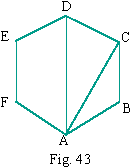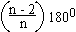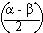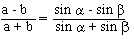Your browser does not support the IFRAME tag.Home MonkeyNotes Printable Notes Digital Library Study Guides Study Smart Parents Tips College Planning Test Prep Fun Zone Help / FAQ How to Cite New Title Request

SUPPLEMENTARY PROBLEMS

1. (a) Solve the following oblique triangle ABC, given

a = 125, Ð A = 540 40' , Ð B = 650 10'

Answer :- b = 139, c = 133, Ð C = 600 10'

(b) a = 320, c = 475, A = 350 20'

Answer :- b = 552, Ð B = 850 30', Ð C = 590 10'

b' = 224, Ð B' = 230 50', Ð C' = 1200 50'

(c) Solve the triangle whose sides are respectively 52.8, 39.3 and 72.1

Answer :- Ð A = 450 44' , Ð B = 320 12', Ð C = 1020 4'

(d) In triangle ABC, a = 54 cms, b = 78 cms and c = 92 cm. Find the greatest angle.

(e) The sides of the triangle are 7 cms, 4 Ö 3 cms and Ö 13 cms. Find the smallest angles.

2. (a) A light house is 10 miles northwest of a dock. A ship leaves the dock at 9 A.M. and steams west at 12 miles / hour. At what time will it be 8 miles from the light house ?

(b) Two forces of 115 1b and 215 1b acting on an object have a resultant of magnitude 275 1b. Find the angle between the directions in which the given forces act .

(c)In the regular hexagon shown. Find the length AC and AD if each side has length 6.23 units. Use the fact that in a regular hexagon, each angle is,(d) Two planes take off from the same air port. The first one flies on a course of 220.10. The second one flies on a course of 154.40. After the first plane flies 362.4 kms, the course from the second plane is 83.50. How far is the second plane from the airport ?Your browser does not support the IFRAME tag.

3. (a) Verify the identity (a - b) cos r /2 = c sin, which is known as Mollweide's formula.

(b) Prove that4. (a) Solve the triangle ABC if a = 12, b = 10 and a = 510

Answer :- b1 = 40.36 25 , b2 = 139.637 g1 = 88.6375, g2 =
-10.6370, c1 = 15.4367. Since negative angle g2 is impossible. There is only one solution. Check it by b < a.

(b) A small electronic component in the shape of a triangle with sides 6.23, 8.146 and 11.392 millimeters. Find the largest angle. Ans :- 104.031 0

**********

Index

3. 1 Solving Right Triangles
3. 2 Law of Cosines
3. 3 Law of Sines
3. 4 The Ambiguous Case of Law of Sines
3. 5 Areas of Triangles
Supplementary Problems

Chapter 4Search: All Products Books Popular Music Classical Music Video DVD Toys & Games Electronics Software Tools & Hardware Outdoor Living Kitchen & Housewares Camera & Photo Cell Phones Keywords: